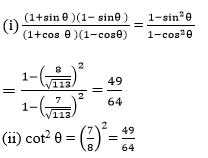Newbie

# If cot θ = 7/8, evaluate : (i) (1 + sin θ)(1 – sin θ)/(1+cos θ)(1-cos θ) . Q.7(1)

• 1

How i solve the question of introduction to trigonometry of ncert class 10 of exercise 8.1. Find the easy way to solve this question no.7(1) properly  and give me the easiest solution. If cot θ = 7/8, evaluate : (i) (1 + sin θ)(1 – sin θ)/(1+cos θ)(1-cos θ) .

Share

1. Let us assume a △ABC in which ∠B = 90° and ∠C = θ

Given:

cot θ = BC/AB = 7/8

Let BC = 7k and AB = 8k, where k is a positive real number

According to Pythagoras theorem in △ABC we get.

AC2 = AB2+BC2

AC2 = (8k)2+(7k)2

AC2 = 64k2+49k2

AC2 = 113k2

AC = √113 k

According to the sine and cos function ratios, it is written as

sin θ = AB/AC = Opposite Side/Hypotenuse = 8k/√113 k = 8/√113 and

cos θ = Adjacent Side/Hypotenuse = BC/AC = 7k/√113 k = 7/√113

Now apply the values of sin function and cos function:• 1